# Maths Meets Art: How Artists Use Geometry And Symmetry To Create A Masterpiece

###### ByRamraj Saini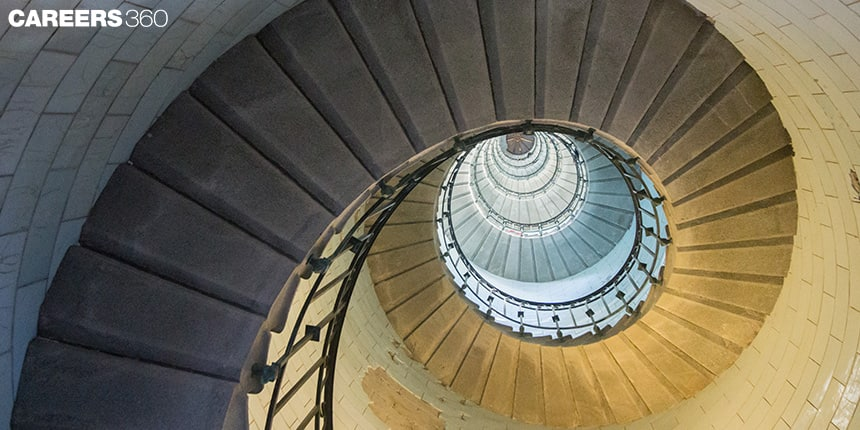###### Synopsis

Art and Maths, do you think they could be interconnected? Yes, of course, the shapes, sizes, patterns, measure of colour, and medium all are concepts of maths that an artist employs to create something beautiful. Let’s take a look at what concepts of maths and geometry are used by artists to create fascinating creations.###### Synopsis

Art and Maths, do you think they could be interconnected? Yes, of course, the shapes, sizes, patterns, measure of colour, and medium all are concepts of maths that an artist employs to create something beautiful. Let’s take a look at what concepts of maths and geometry are used by artists to create fascinating creations.

Maths and art may seem like two completely unrelated subjects, but they are actually deeply interconnected. Both maths and art involve the use of patterns, shapes, and designs to create something beautiful or meaningful. In school, studying maths and art helps students develop problem-solving skills, critical thinking, and enhance their creativity. While maths teaches students how to think logically and analytically, art satiates their creative expression. By studying both subjects, students learn how to combine these different ways of creative thinking and problem solving to create something new.

Many fields, such as architecture, engineering, graphic design, and computer science, require a strong foundation in both maths and art. Understanding the principles of maths and art can also be useful for fields in professions such as advertising, marketing, and product design. Now let's look at mathematics concepts which are useful in art work.

## Geometric Shapes And Patterns

One of the most obvious ways in which maths and art are related is through the use of geometric shapes and patterns. Artists throughout history have used geometric shapes and patterns to create art that is both visually striking and mathematically precise with help of mathematical principles, such as the golden ratio.

### Fibonacci Ratio

The Fibonacci ratio, also known as the golden ratio, is a mathematical concept that is often used in art and design to create visually pleasing and harmonious compositions. The golden ratio is approximately 1.618 and is represented by the Greek letter phi (φ). It can be found in many natural phenomena such as the spiral patterns in seashells and the branching patterns in trees.

In art and design, the golden ratio is often used to create a sense of balance and harmony in a composition. This is achieved by dividing a piece of art or design into parts that are in proportion to the golden ratio. For example, an artist might divide a canvas into two parts, one that is 1.618 times larger than the other. The artist could then place the main subject of the painting within the larger section, creating a sense of balance and harmony in the composition.

## Use Of Perspective In Art

In art, perspective is used to create the illusion of depth and three-dimensional space on a two-dimensional surface. This is achieved through the use of mathematical principles, such as the rules of point and linear perspective. Similarly, in photography too, the use of depth of field and aperture is based on mathematical principles.

### Point Perspective

One-point perspective is a technique where parallel lines in a scene converge at a single "vanishing point" to create the illusion of depth. This technique is commonly used in painting and drawing to create a more three-dimensional feel. This maths concept can be understood using the following art.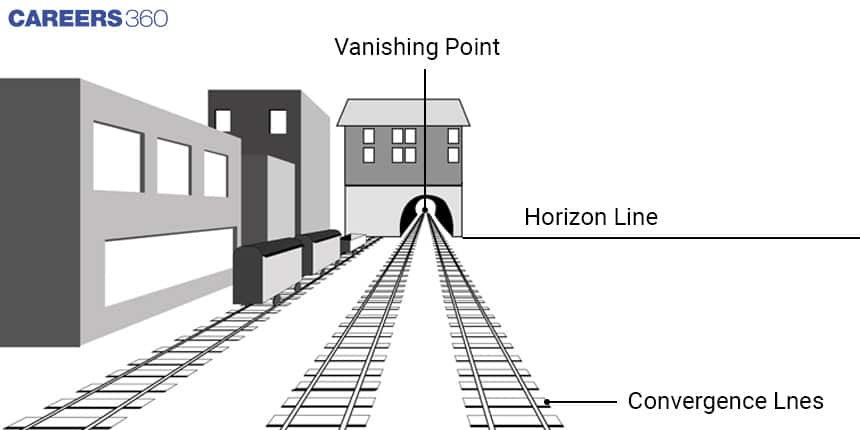### Linear Perspective

Linear perspective in art refers to the technique of using mathematical principles to create the illusion of depth and distance in a two-dimensional artwork. This can be achieved through the use of converging lines, overlapping shapes, and variations in size and colour. Understanding and utilising linear perspective can greatly enhance the realism and impact of a piece of art

### Fractal Geometry

Fractal geometry is a branch of mathematics that deals with the study of shapes and patterns that exhibit self-similarity, meaning that they look similar at different scales. These shapes and patterns are known as fractals.

Fractals are geometric shapes that repeat at different scales and can be used to generate complex shapes with minimal information. Examples of fractals can be found in our surroundings such as clouds, mountains, and coastlines, and they can also be generated using mathematical algorithms. This technique is often used in computer generated imagery (CGI) to create intricate designs. Following art can help students to understand fractal geometry.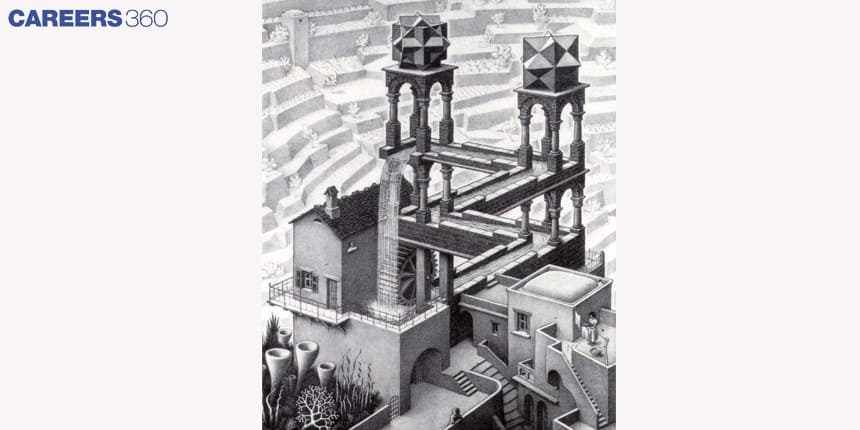Fractal geometry is important for class 12 students to study as it has a wide range of real-world applications, such as in computer graphics, digital image processing, and many other fields of science and engineering. Understanding fractals and their properties can help students to develop problem-solving and analytical skills that are valuable in many fields.

Additionally, the use of mathematical algorithms and computer programming to create art, commonly known as generative art, is becoming increasingly popular. This type of art is created using mathematical formulas and computer algorithms to generate images, animations, and even music. This is a great example of how maths and art can intersect and create something new.

• Branches of science
• Mathematics
• Geometry
• Fractal
• Perspective (graphical)
• Pattern
• Mathematics and art
• Composition (visual arts)
• Golden ratio

## Careers360 helping shape your Career for a better tomorrow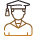#### 250M+

Students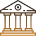#### 30,000+

Colleges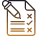#### 500+

Exams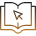E-Books